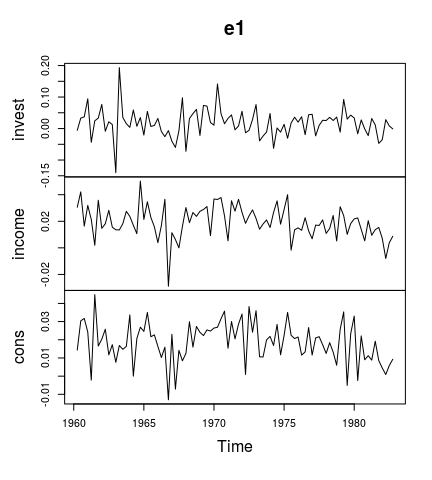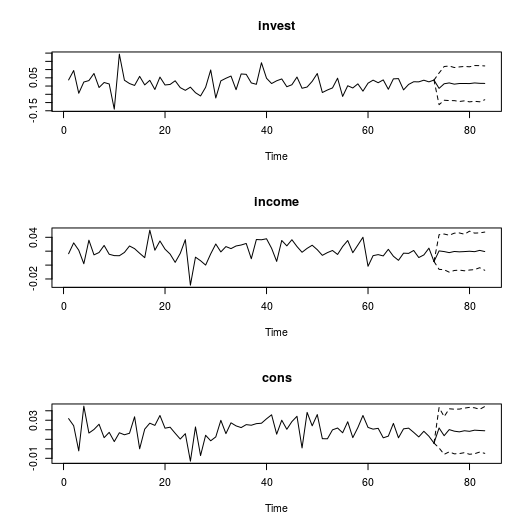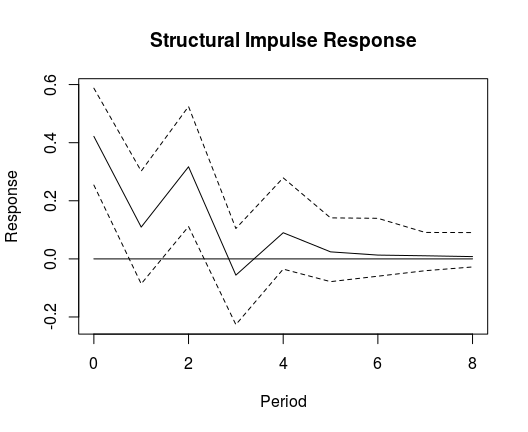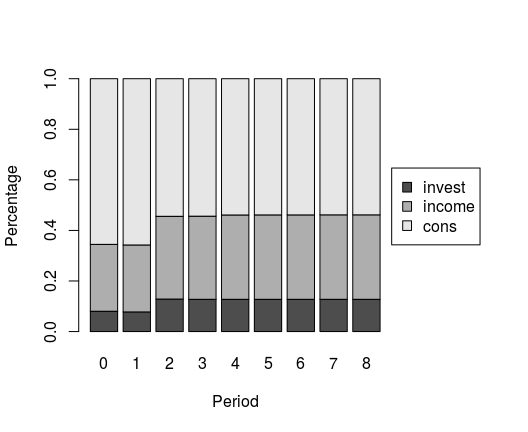# Bayesian Structural Vector Autoregression

## Introduction

This vignette illustrates the use of the bvartools package for Bayesian inference of structrual VAR models. For this purpose the dataset E1 from Lütkepohl (2007) is used. It contains data on West German fixed investment, disposable income and consumption expenditures in billions of DM from 1960Q1 to 1982Q4.

library(bvartools)

data("e1")
e1 <- diff(log(e1))

plot(e1) # Plot the seriesThe considered model is a structural VAR(2) with a constant term and structural coefficients in the errors:

$y_t = v + \sum_{i = 1}^{2} A_i y_{t-i} + A_0^{-1} u_t.$

$$y_t$$ is a $$K$$-dimensional vector of endogenous variables in period $$t$$, $$A_i$$ is a $$K \times K$$ coefficient matrix of lagged values of $$y_t$$, $$u_t \sim \Sigma$$ is an error term with zero mean and diagonal variance matrix $$\Sigma$$. $$A_0$$ is a lower triangular matrix with ones on the main diagonal.

data <- gen_var(e1, p = 2, deterministic = "const")

y <- data$Y[, 1:73] x <- data$Z[, 1:73]

## Estimation

The following code is a Gibbs sampler for a Bayesian structural VAR model with non-informative priors.

# Reset random number generator for reproducibility
set.seed(1234567)

iter <- 10000 # Number of iterations of the Gibbs sampler
burnin <- 5000 # Number of burn-in draws
store <- iter - burnin

t <- ncol(y) # Number of observations
k <- nrow(y) # Number of endogenous variables
m <- k * nrow(x) # Number of estimated coefficients
k0 <- k * (k - 1) / 2 # Number of structural coefficients

# Set (uninformative) priors
a_mu_prior <- matrix(0, m) # Vector of prior parameter means
a_v_i_prior <- diag(0, m) # Inverse of the prior covariance matrix

a0_mu_prior <- matrix(0, k0) # Vector of prior parameter means
a0_v_i_prior <- diag(0, k0) # Inverse of the prior covariance matrix

sigma_df_prior <- 0 # Prior degrees of freedom
sigma_scale_prior <- rep(0, k)
sigma_df_post <- t + sigma_df_prior

# Initial values
a0 <- diag(1, k)
omega_i <- rWishart(1, t, solve(tcrossprod(y)))[,, 1]
omega <- solve(omega_i)
sigma_i <- diag(1, k)
diag(sigma_i) <- diag(omega_i)
sigma <- solve(sigma_i)

# Data containers for posterior draws
draws_a <- matrix(NA, m, store)
draws_a0 <- matrix(NA, k^2, store)
draws_omega <- matrix(NA, k^2, store)

# Start Gibbs sampler
for (draw in 1:iter) {
# Draw conditional mean parameters
a <- post_normal(y, x, omega_i, a_mu_prior, a_v_i_prior)

# Structural coefficients
y_tilde <- y - matrix(a, k) %*% x # Obtain residuals
for (j in 2:k) {
# Preparing the data
y_tilde_temp <- matrix(y_tilde[j, ], 1)
x0_temp <- matrix(-y_tilde[1:(j - 1),], j - 1)
a0_sigma_i_temp <-  matrix(sigma_i[j, j])
pos_temp <- (j - 1) * (j - 2) / 2 + 1:(j - 1)
mu_temp <- matrix(a0_mu_prior[pos_temp,])
v_i_temp <- matrix(a0_v_i_prior[pos_temp, pos_temp], j - 1)

# Draw structural coefficients
a0_temp <- post_normal(y = y_tilde_temp, x = x0_temp, sigma_i = a0_sigma_i_temp,
a_prior = mu_temp, v_i_prior = v_i_temp)

# Update A0 matrix
a0[j, 1:(j - 1)] <- a0_temp
}

# Draw variances
y_star <- a0 %*% y_tilde
sigma_scale_post <- sigma_scale_prior + rowSums(y_star^2)
for (j in 1:k) {
sigma_i[j, j] <- rgamma(1, shape = sigma_df_post / 2, rate = sigma_scale_post[j] / 2)
}
sigma <- solve(sigma_i)

a0_i <- solve(a0)
omega <- a0_i %*% tcrossprod(sigma, a0_i)
omega_i <- solve(omega)

# Store draws
if (draw > burnin) {
draws_a[, draw - burnin] <- a
draws_a0[, draw - burnin] <- a0
draws_omega[, draw - burnin] <- sigma
}
}

After the Gibbs sampler has finished, point estimates can be obtained as the mean of the posterior draws:

A <- rowMeans(draws_a) # Obtain means for every row
A <- matrix(A, k) # Transform mean vector into a matrix
A <- round(A, 3) # Round values
dimnames(A) <- list(dimnames(y)[], dimnames(x)[]) # Rename matrix dimensions

A # Print
#>        invest.1 income.1 cons.1 invest.2 income.2 cons.2  const
#> invest   -0.319    0.144  0.954   -0.161    0.112  0.926 -0.016
#> income    0.043   -0.155  0.290    0.049    0.019 -0.005  0.016
#> cons     -0.002    0.223 -0.263    0.034    0.354 -0.020  0.013

The results are similar to the results of the reduced form model in section 3.2.3 of Lütkepohl (2007). The means of the structural coefficients are

A0 <- rowMeans(draws_a0) # Obtain means for every row
A0 <- matrix(A0, k) # Transform mean vector into a matrix
A0 <- round(A0, 3) # Round values
dimnames(A0) <- list(dimnames(y)[], dimnames(y)[]) # Rename matrix dimensions

solve(A0) # Print
#>          invest income cons
#> invest 1.000000  0.000    0
#> income 0.035000  1.000    0
#> cons   0.058875  0.425    1

## bvar objects

The bvar function can be used to collect relevant output of the Gibbs sampler into a standardised object, which can be used by further functions such as predict to obtain forecasts, irf for impulse respons analysis or fevd for forecast error variance decomposition.

bvar_est <- bvar(y = y, x = x, A = draws_a[1:18,], C = draws_a[19:21, ],
A0 = draws_a0, Sigma = draws_omega)

Posterior draws can be thinned with function thin:

bvar_est <- thin(bvar_est, thin = 5)

## Forecasts

Forecasts with credible bands can be obtained with the function predict. If the model contains deterministic terms, new values can be provided in the argument new_D. If no values are provided, the function sets them to zero. The number of rows of new_D must be the same as the argument n.ahead.

If draws of $$A_0$$ are contained in the bvar object, the credible bands are based on $$A_0^{-1} \Sigma A_0^{-1\prime}$$.

bvar_pred <- predict(bvar_est, n.ahead = 10, new_D = rep(1, 10))

plot(bvar_pred)## Impulse responses

Structrual impulse respones can be obtained by setting type = "sir" in the irf function.

SIR <- irf(bvar_est, impulse = "income", response = "cons", n.ahead = 8, type = "sir")

plot(SIR, main = "Structural Impulse Response", xlab = "Period", ylab = "Response")Although the values differ, the form of the SIR is the same as for an orthogonalised impulse respons obtained from a reduced form VAR, because of the lower triangular structur of the structural coefficient matrix.

## Structrual forecast error variance decomposition

Structrual forecast error variance decomposition (SFEVD) can be done by setting type = "sir" in the fevd function.

SFEVD <- fevd(bvar_est, response = "cons", n.ahead = 8, type = "sir")

plot(SFEVD, main = "Structrual FEVD of consumption")The form of the SFEVD is the same as for an FEVD that is based on orthogonalised impulse responses obtained from a reduced form VAR, because of the lower triangular structur of the structural coefficient matrix.